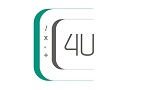﻿ Set Difference | Difference of Two Sets Calculator | Calculators4U# Set Difference - Difference of Two Sets Calculator

In the set operations, let's calculate set difference. The difference of two set A and set B, denoted by A - B, is the set that contains exactly all elements in A but not in B.